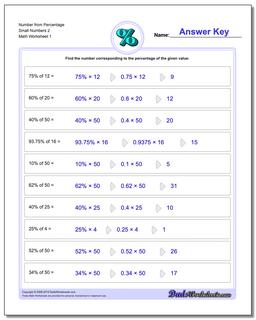# Math Worksheets: Percentages: Percentages: Number from Percentage Small Numbers 2## Number from Percentage Small Numbers 2

PropertyValue
DescriptionNumber from Percentage Small Numbers 2: Percent worksheets where students are given a whole number and a percentage, and must calculate the fraction of the whole represented.
Resource TypeWorksheet